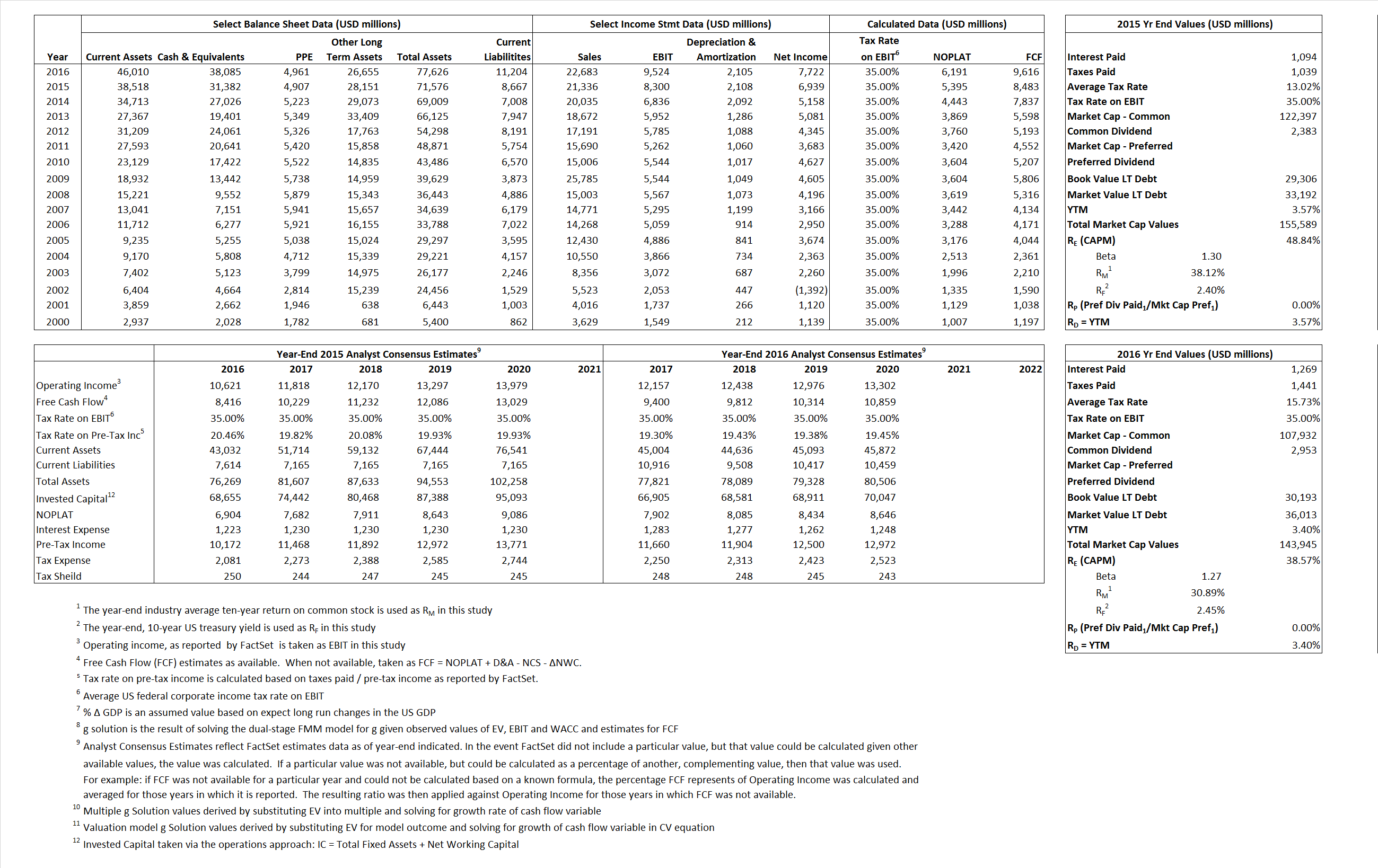# Amgen Inc.

## Analyst Listing

The following analysts provide coverage for the subject firm as of May 2016:

 Broker Analyst Analyst Email Credit Suisse Alethia Young alethia.young@credit-suisse.com Raymond James Christopher J. Raymond chris.raymond@raymondjames.com Cowen & Company Eric Schmidt eric.schmidt@cowen.com Jefferies Eun K. Yang eyang@jefferies.com Leerink Partners Geoffrey C. Porges geoffrey.porges@leerink.com BTIG Hartaj Singh hsingh@btig.com Wells Fargo Securities Jim Birchenough jim.birchenough@wellsfargo.com William Blair John Sonnier jsonnier@williamblair.com Piper Jaffray Joshua Schimmer joshua.e.schimmer@pjc.com BMO Capital Markets M. Ian Somaiya ian.somaiya@bmo.com Evercore ISI Mark Schoenebaum mark.schoenebaum@evercoreisi.com RBC Capital Markets Michael J. Yee michael.yee@rbccm.com Atlantic Equities Steve Chesney s.chesney@atlantic-equities.com

## Primary Input Data## Derived Input Data

### Equational Form

Net Operating Profit Less Adjusted Taxes NOPLAT 5,395 6,191$NOPLAT\, =\, EBIT\, x\, (1 \,-\, Avg \,\,Tax\,\, Rate\,\, on\,\, EBIT)$
Free Cash Flow FCF 8,483 9,616$FCF\,=NOPLAT\,+\,Non-Cash\,Expenses-\Delta NWC\,-\,NCS$
Tax Shield TS 142 200$TS\,=\,Interest\,\,Paid\,\,x\,\, Avg \,\,Tax\,\,Rate\,\, on\,\, Pre-Tax\,\, Income$
Invested Capital IC 62,909 66,422$IC\,=\,Fixed\,\,Operating\,\,Assets\,\,+\,\,Net\,\, Working\,\, Capital$
Return on Invested Capital ROIC 8.58% 9.32%$ROIC\,=\,\frac { NOPLAT }{ IC }$
Net Investment NetInv 3,016 5,618$NetInv\,=\,{ {IC}_{1}}-{{IC}_{0}}+Depreciation$
Investment Rate IR 55.90% 90.75%$IR\,=\,\frac {NetInv}{NOPLAT}$
Weighted Average Cost of Capital
WACCMarket 39.08% 29.64%$WACC\,=\,\frac { E }{ V } { R }_{ E }\,+\,\frac { P }{ V } { R }_{ P }\,+\,\frac { D }{ V } { R }_{ D }\left( 1- Avg\,\, Tax\,\,Rate\,\,on\,\,Pre-Tax\,\,Income \right)$
WACCBook  9.71%  8.73%
Enterprise value
EVMarket 124,207  105,860$EV\,=\,Market\,\,Cap\,\,Equity\,+\,\,Long\,\,Term\,\,Debt\,-\,Cash$
EVBook  120,321  100,040
Long-Run Growth
g = IR x ROIC
4.79%  8.46% Long-run growth rates of the income variable are used in the Continuing Value portion of the valuation models.
g = %$\Delta$ GDP  2.50%  2.50%
Margin from Operations M 38.90%  41.99%$M\,\,=\,\,\frac{EBIT}{SALES}$
Depreciation/Amortization Rate D 20.25%  18.10%$D\,\,=\,\,\frac{D+A}{EBITDA}$

## Valuation Multiple Outcomes

The outcomes presented in this study are the result of original input data, derived data, and synthesized inputs.

### model g solution

12/31/2015 12/31/2016 12/31/2015 12/31/2016 12/31/2015 12/31/2016

EV/SALES$\frac {EV}{Sales} \,= \,\frac{ROIC\, -\, g}{ROIC\,(WACC\,-\,g)}\,(1\,-\,T)\,(M)$

5.82  4.67  70.39%  63.85%  43.14%  35.44%

EV/EBITDA$\frac {EV}{EBITDA} \,= \,\frac{ROIC\, -\, g}{ROIC\,(WACC\,-\,g)}\,(1\,-\,T)\,(1\,-\,D)$

11.93 9.10 70.39% 63.85% 43.14% 35.44%

EV/NOPLAT$\frac {EV}{NOPLAT} \,= \,\frac{ROIC\, -\, g}{ROIC\,(WACC\,-\,g)}$

23.02 17.10 70.39% 63.85% 43.14% 35.44%

EV/FCFOPS$\frac {EV}{FCF_{OPS}} \,= \,\frac{ROIC\, -\, g}{ROIC\,(WACC\,-\,g)}\,(1\,-\,T)$

14.64 11.01 70.39% 63.85% 43.14% 35.44%

EV/EBIT$\frac {EV}{EBIT} \,= \,\frac{ROIC\, -\, g}{ROIC\,(WACC\,-\,g)}\,(1\,-\,T)$

14.96 11.12 70.39% 63.85% 43.14% 35.44%

EV/IC$\frac {EV}{IC} \,= \,\frac{ROIC\, -\, g}{WACC\,-\,g}$

1.97 1.59 70.39% 63.85% 43.14% 35.44%